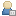###Show Posts

This section allows you to view all posts made by this member. Note that you can only see posts made in areas you currently have access to.

### Messages - tzafrir

Pages: 1 ... 7 8 
121
##### General Board / Re: Add or delete an element from a diagram
« on: December 30, 2015, 03:55:03 am »
Hi,

Add element to diagram was resolved but the requested element for deletion is not deleted from diagram.
What I see is:
diagram.DiagramObjects.DeleteAt(index,true) does delete the element,
as mentioned above i can see the size of diagramObjects is reduced by 1.
I removed the diagram.DiagramObjects.Refresh().
and run it with and without diagram.Update() API call.
both cases element stays on the diagram.

I also run a test that add a new element to a diagram and it is shown when closing and opening the diagram.
(So diagramObjects are getting refreshed but without the deletion)
Any other ideas?

122
##### General Board / Re: Add or delete an element from a diagram
« on: December 29, 2015, 05:35:26 am »
Sorry for the confusing sample, in my code I use someElement, this was a typo when I created the mail
Anyway I changed the addNew to get the position and still getting this exception:

{
EA.Diagram diagram = repository.GetCurrentDiagram();
EA.DiagramObject a = (EA.DiagramObject) diagram.DiagramObjects.AddNew("l=200;r=400;t=200;b=600;", "");
a.ElementID = someElement.ElementID;
a.Update(); //The update is throwing an exception
diagram.Update();
}

For the delete function, i saw that the element was deleted by using the count method but the diagram was not updated in model (with the update and refresh methods)
private deleteFromDiagram(EA.Repository repository,EA.Element someElement)
{
EA.Diagram diagram = repository.GetCurrentDiagram();

int i = diagram.DiagramObjects.Count; //2
diagram.DiagramObjects.DeleteAt(1, true); //delete the second element in diagram
i = diagram.DiagramObjects.Count; //1
diagram.DiagramObjects.Refresh();
diagram.Update();
}

The sample code I saw in the forum looks pretty much like mine, but something is still missing...

123
##### General Board / Re: Add or delete an element from a diagram
« on: December 28, 2015, 07:33:58 am »I know it should be simple but I am missing something basic here:

For the Add method all I want to do is get an element and put it on the diagram

this simplify what I wrote:
{
EA.Diagram diagram = repository.GetCurrentDiagram();
a.ElementID = selected.ElementID;
a.Update(); //The update is throwing an exception
diagram.Update();
}
In debugger I see the element Id is getting updated, but the Update throws an exception.

For the delete function, all it should do is get element an element which is on the diagram and delete it:
private deleteFromDiagram(EA.Repository repository,EA.Element someElement)
{
EA.Diagram diagram = repository.GetCurrentDiagram();
short id = (short)selected.ElementID;
diagram.DiagramObjects.DeleteAt(id, true); //this line throws an exception for a valid element
diagram.Update();
}
Again, what is wrong with this delete?

Tzafrir

124
##### General Board / Re: Add or delete an element from a diagram
« on: December 27, 2015, 06:21:17 pm »
I tried to use those APIs, but none of them work as I expect. I am probably missing something here.

I will use a sample code to be more clear:

EA.Diagram diagram = repository.GetCurrentDiagram();
diagramObj.ElementID = someElement.ElementID;
diagram.Update();

For the delete API:
short id = (short)someElement.ElementID;
diagram.DiagramObjects.DeleteAt(id,false);
(This is throwing an exception on an element that is on the diagram)

I think I used them both properly, can you assist me with what is wrong?

Tzafrir

125
##### General Board / Add or delete an element from a diagram
« on: December 26, 2015, 08:32:01 am »
Hi,

I have an element that i want to add to a specific diagram through the API.
And I have an element that I want to remove from the diagram.
I could not find an API that does that.
Does anyone know how this can be achieved?

Thanks,
Tzafrir

126
##### Automation Interface, Add-Ins and Tools / Adding element to diagram and remove element from diagram
« on: December 26, 2015, 08:31:10 am »
Hi,

I have an element that i want to add to a specific diagram through the API.
And I have an element that I want to remove from the diagram.
I could not find an API that does that.
Does anyone know how this can be achieved?

Thanks,
Tzafrir

127
##### Suggestions and Requests / List of tables sql queries can be run on
« on: December 25, 2015, 04:33:52 am »
Hi,

Can anyone post a list of tables and there fields (or where there is a link that it can be found at).

Thanks,
Tzafrir

Pages: 1 ... 7 8